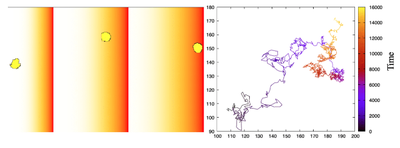# One Random Walker

##### Persistent Identifier

Unbiased and biased random walkers

## Introduction

We can simulate the swimming motion of a cell, such as a bacterium, by interspersing short periods of directed motion with random turns or random scrambling of the cell’s velocity.

## Description

A cell is started at the center of the domain. At fixed time increments, the walker changes its direction of motion. Its velocity vector is then recomputed in an Event as a vector with random $x$ and $y$ components. A bias in the move direction can be introduced by setting BiasOnOff to $0$ (off) or $1$ (on).

## Results

Sample trajectories of a random walker with bias (right, BiasOnOff $= 1$) and without bias (left, BiasOnOff $= 0$) towards the $y$ direction. At fixed time increments, the walker changes its direction of motion, with new velocity vector recomputed with random $x$ and $y$ components. Time along the trajectories is color-coded from black to yellow.A random walker in 2D: a cell is started at the center of the domain. Produced with OneRandomWalker_main.xml.Left: Three sample snapshots of the cell and chemical concentration. Right: Cell trajectory over $15000\ \mathrm{MCS}$. The cell spends most of its time in the high $c$ region, as its run length drops and frequency of turning increases with $c$. Produced with OneRandomWalkerWithChemicalBias.xml.

## Model

Get this model via:

•  Download: OneRandomWalker_main.xml
• XML Preview

<?xml version='1.0' encoding='UTF-8'?>
<MorpheusModel version="4">
<Description>
<Title>OneRandomWalker</Title>
<Details>Full title:		One Random Walker
Authors:		L. Edelstein-Keshet
Contributors:	Y. Xiao
Date:		10.05.2022
Model ID:		https://identifiers.org/morpheus/M2003
File type:		Main model
Reference:		L. Edelstein-Keshet: Mathematical Models in Cell Biology
Comment:		A Random walker in 2D: a cell is started at the center of the domain. At fixed time increments, the walker changes its direction of motion. Its velocity vector is then recomputed as a vector with random x and y components. A bias in the move direction can be introduced by setting BiasOnOff to 0 (Off) or 1 (On). Thanks: Uses some aspects of example RunAndTumble (Morpheus Model ID: M0025, https://identifiers.org/morpheus/M0025) provided in Morpheus.</Details>
</Description>
<Global/>
<Space>
<Lattice class="hexagonal">
<Size symbol="size" value="200, 200, 0"/>
<Neighborhood>
<Order>1</Order>
</Neighborhood>
<BoundaryConditions>
<Condition type="periodic" boundary="x"/>
<Condition type="periodic" boundary="y"/>
</BoundaryConditions>
</Lattice>
<SpaceSymbol symbol="space"/>
</Space>
<Time>
<StartTime value="0"/>
<StopTime value="10000"/>
<TimeSymbol symbol="time"/>
</Time>
<CellTypes>
<CellType class="biological" name="Walker">
<VolumeConstraint target="200" strength="1"/>
<ConnectivityConstraint/>
<PropertyVector symbol="move_dir" value="0.0, 0.0, 0.0"/>
<PropertyVector symbol="displacement" value="0.0, 0.0, 0.0"/>
<PropertyVector symbol="velocity" value="0.0, 0.0, 0.0"/>
<PropertyVector symbol="BiasDirection" name="spatial cue direction" value="0, 1.0, 0.0"/>
<Property symbol="BiasOnOff" name="Turn cue on (1) or off (0)" value="0.0"/>
<Property symbol="run_time" name="run duration" value="5.0"/>
<Property symbol="last_turn_time" name="last random event" value="0"/>
<DirectedMotion direction="20*move_dir+BiasDirection*BiasOnOff" strength="1"/>
<Event trigger="when-true" time-step="5" name="Change direction">
<Condition>time >= last_turn_time+ run_time</Condition>
<Rule symbol-ref="last_turn_time" name="Time of last random event">
<Expression>time</Expression>
</Rule>
<VectorRule symbol-ref="move_dir" name="new direction of motion">
<Expression>rand_uni(-1,1), rand_uni(-1,1) , 1</Expression>
</VectorRule>
</Event>
</CellType>
<CellType class="medium" name="medium"/>
</CellTypes>
<CPM>
<Interaction>
<Contact type1="Walker" type2="medium" value="4"/>
<Contact type1="Walker" type2="Walker" value="10"/>
</Interaction>
<MonteCarloSampler stepper="edgelist">
<MCSDuration value="1"/>
<Neighborhood>
<Order>1</Order>
</Neighborhood>
<MetropolisKinetics temperature="0.6"/>
</MonteCarloSampler>
<ShapeSurface scaling="norm">
<Neighborhood>
<Distance>2.5</Distance>
</Neighborhood>
</ShapeSurface>
</CPM>
<CellPopulations>
<Population type="Walker" size="1" name="Random Walker">
<!--    <Disabled>
<Cell id="1" name="1">
<Nodes>100,100,0</Nodes>
</Cell>
</Disabled>
-->
<InitCircle number-of-cells="1" random_displacement="5.0" mode="random">
</InitCircle>
</Population>
</CellPopulations>
<Analysis>
<Gnuplotter time-step="200" decorate="false">
<Terminal name="png"/>
<Plot>
<Cells value="cell.id"/>
<CellArrows orientation="20*velocity" center="midpoint" style="1"/>
</Plot>
</Gnuplotter>
<Logger time-step="10">
<Input>
<Symbol symbol-ref="cell.center.x"/>
<Symbol symbol-ref="cell.center.y"/>
</Input>
<Output>
<TextOutput/>
</Output>
<Plots>
<Plot time-step="5000">
<Style line-width="1.5" style="lines"/>
<Terminal terminal="png"/>
<X-axis>
<Symbol symbol-ref="cell.center.x"/>
</X-axis>
<Y-axis>
<Symbol symbol-ref="cell.center.y"/>
</Y-axis>
<Color-bar>
<Symbol symbol-ref="time"/>
</Color-bar>
</Plot>
</Plots>
</Logger>
<DisplacementTracker time-step="10" name="data" celltype="Walker"/>
<ModelGraph format="svg" reduced="false" include-tags="#untagged"/>
</Analysis>
</MorpheusModel>